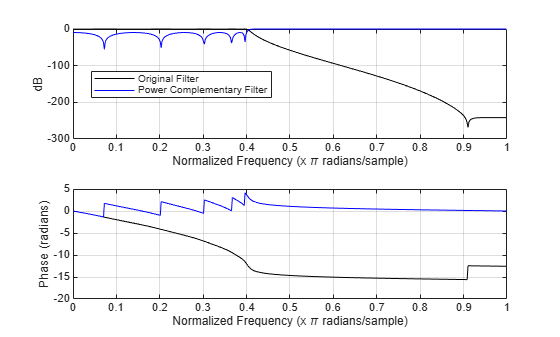# cl2tf

Convert coupled allpass lattice to transfer function form

## Syntax

[b,a] = cl2tf(k1,k2)
[b,a] = cl2tf(k1,k2,beta)
[b,a,bp] = cl2tf(k1,k2)
[b,a,bp] = cl2tf(k1,k2,beta)

## Description

[b,a] = cl2tf(k1,k2) returns vectors of coefficients b and a when k1 and k2 are real vectors. b is the vector of coefficients corresponding to the numerator of the transfer function H(z). a is the vector of coefficients corresponding to the denominator of the transfer function H(z). k1 and k2 are real vectors corresponding to denominators of the allpass filters H1(z) and H2(z). This is provided in the transfer function:$H\left(z\right)=B\left(z\right)/A\left(z\right)=\frac{1}{2}\left[H1\left(z\right)+H2\left(z\right)\right]$
[b,a] = cl2tf(k1,k2,beta) returns the vectors of coefficients b and a corresponding to the numerator and denominator, respectively, of the transfer function H(z), where k1, k2, and beta are complex vectors.$H\left(z\right)=B\left(z\right)/A\left(z\right)=\frac{1}{2}\left[-\left(\overline{\beta }\right)\cdot H1\left(z\right)+\beta \cdot H2\left(z\right)\right]$
[b,a,bp] = cl2tf(k1,k2) also returns the vector bp of real coefficients corresponding to the numerator of the power-complementary filter G(z), where k1 and k2 are real vectors.$G\left(z\right)=Bp\left(z\right)/A\left(z\right)=\frac{1}{2}\left[H1\left(z\right)-H2\left(z\right)\right]$
[b,a,bp] = cl2tf(k1,k2,beta) also returns the vector of coefficients bp of possibly complex coefficients corresponding to the numerator of the power complementary filter G(z), where k1, k2, and beta are complex.$G\left(z\right)=Bp\left(z\right)/A\left(z\right)=\frac{1}{{2}_{j}}\left[-\left(\overline{\beta }\right)\cdot H1\left(z\right)+\beta \cdot H2\left(z\right)\right]$

## Examples

collapse all

Compute the reflection coefficient using tf2cl function.

[b,a] = cheby1(10,.5,.4); [k1,k2,beta] = tf2cl(b,a);

Construct the original and the power-complementary filters.

[num,den,numpc] = cl2tf(k1,k2,beta); [h,w] = freqz(num,den); hpc = freqz(numpc,den);

Plot the frequency response.

subplot(211) plot(w./pi,20*log10(abs(h)),'k') hold on; grid on; plot(w./pi,20*log10(abs(hpc)),'b') xlabel('Normalized Frequency (x \pi radians/sample)'); ylabel('dB'); legend('Original Filter','Power Complementary Filter',... 'Location','best'); subplot(212) plot(w./pi,unwrap(angle(h)),'k') hold on; grid on; xlabel('Normalized Frequency (x \pi radians/sample)'); ylabel('Phase (radians)'); plot(w./pi,unwrap(angle(hpc)),'b')## Input Arguments

collapse all

Reflection coefficients corresponding to allpass lattice structure of H1(z), specified as a real- or complex-valued vector.

Data Types: single | double
Complex Number Support: Yes

Reflection coefficients corresponding to allpass lattice structure of H2(z), specified as a real- or complex-valued vector.

Data Types: single | double
Complex Number Support: Yes

Complex scalar of magnitude 1.

Data Types: single | double
Complex Number Support: Yes

## Output Arguments

collapse all

Coefficients of the numerator of the transfer function H(z), returned as a real- or complex-valued vector.

Data Types: double
Complex Number Support: Yes

Coefficients of the denominator of the transfer function H(z), returned as a real- or complex-valued vector.

Data Types: double
Complex Number Support: Yes

Coefficients of the numerator of the power-complementary filter G(z), returned as a real- or complex-valued vector.

Data Types: double
Complex Number Support: Yes

 Mitra, Sanjit Kumar, and James F. Kaiser, eds. Handbook for Digital Signal Processing. New York: Wiley, 1993.

 Vaidyanathan, P. P. Multirate Systems and Filter Banks . Prentice-Hall Signal Processing Series. Englewood Cliffs, N.J: Prentice Hall, 1993. CloseDeleteEdit

Watch now